# Mazur-Orlicz theorem

(diff) ← Older revision | Latest revision (diff) | Newer revision → (diff)

A sequence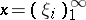is said to be summable toby a methodgiven by an infinite matrix, if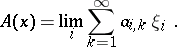Letbe the set of all sequences summables by a method. Such a method is said to be convergence preserving ifcontains all convergent sequences (it is not assumed, however, that for a convergent sequence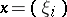one has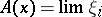; if the latter holds,is called a permanent summability method). For a convergence-preserving methodthere is a well-defined quantity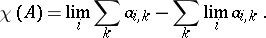Letandbe convergence-preserving methods with, and assume that for each convergent sequenceone has. Then the Mazur–Orlicz theorem is usually given to the following statement: If every bounded sequence inis in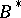, then also for these sequences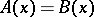([a3], Thm. 2; see also [a1] and [a2]).

A related result is as follows. Ifis a convergence-preserving method, then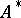contains an unbounded sequence if either of the following is satisfied ([a3], Thm. 7):

i);

ii)andcontains a bounded divergent sequence. A permanent methodis said to be perfectly inconsistent if for each divergent sequenceinthere is a permanent methodwithand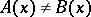.

A permanent methodis perfectly inconsistent if and only if every sequence inis either convergent or unbounded ([a3], Thm. 10).

S. Mazur and W. Orlicz also worked also in functional analysis; e.g., the Banach–Steinhaus theorem for-spaces (see Fréchet topology) is due to them.

How to Cite This Entry:
Mazur-Orlicz theorem. Encyclopedia of Mathematics. URL: http://encyclopediaofmath.org/index.php?title=Mazur-Orlicz_theorem&oldid=12108
This article was adapted from an original article by W. Zelazko (originator), which appeared in Encyclopedia of Mathematics - ISBN 1402006098. See original article